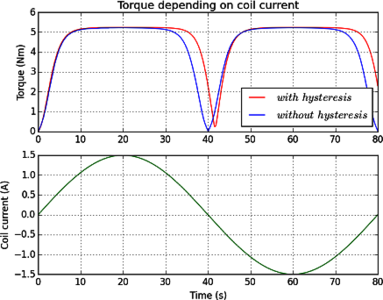# 论文和技术资料

## Simulation of a Rotary Magnetorheological Damper

D. Harder , L. Fromme , R. Naumann ,
 University of Applied Sciences Bielefeld, Department of Engineering Sciences and Mathematics, Bielefeld, Germany
 University of Applied Sciences Bielefeld, ISyM – Institute of System Dynamics and Mechatronics, Bielefeld, Germany

This paper presents a simulation model of a rotary damper filled with a magnetorheological fluid (MRF). The most important characteristic of the MRF is the variable viscosity, which can be controlled by an external magnetic field. In the simulation model, the fluid is described as a Bingham fluid model which is coupled to an electromagnetic field simulation to analyze the damping characteristic in dependence on the coil current. For the calculation of the shear rate the angular velocity of the disk is needed, which can be computed by solving an additional ordinary differential equation. The numerical results show the expected behavior of the damper, i.e. a hysteresis in the damping characteristic.